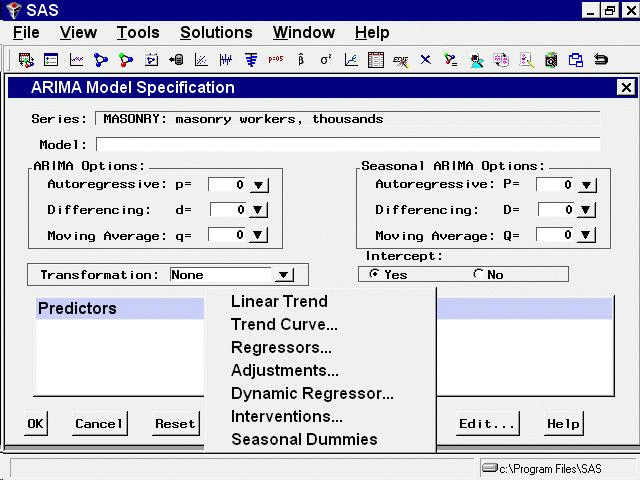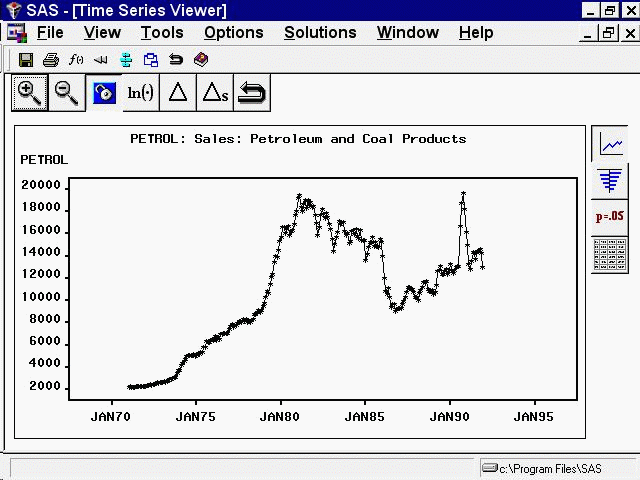# Using Predictor Variables

Forecasting models predict the future values of a series by using two sources of information: the past values of the series and the values of other time series variables. Other variables used to predict a series are called predictor variables.

Predictor variables that are used to predict the dependent series can be variables in the input data set, such as regressors and adjustment variables, or they can be special variables computed by the system as functions of time, such as trend curves, intervention variables, and seasonal dummies.

You can specify seven different types of predictors in forecasting models by using the ARIMA Model or Custom Model Specification windows. You cannot specify predictor variables with the Smoothing Model Specification window.

Figure 50.1 shows the menu of options for adding predictors to an ARIMA model that is opened by clicking the `Add` button. The Add menu for the Custom Model Specification menu is similar.These types of predictors are as follows.

Linear Trend

adds a variable that indexes time as a predictor series. A straight line time trend is fit to the series by regression when you specify a linear trend.

Trend Curve

provides a menu of various functions of time that you can add to the model to fit nonlinear time trends. The Linear Trend option is a special case of the Trend Curve option for which the trend curve is a straight line.

Regressors

allows you to predict the series by regressing it on other variables in the data set.

allows you to specify other variables in the data set that supply adjustments to the forecast.

Dynamic Regressor

allows you to select a predictor variable from the input data set and specify a complex model for the way that the predictor variable affects the dependent series.

Interventions

allows you to model the effect of special events that "intervene" to change the pattern of the dependent series. Examples of intervention effects are strikes, tax increases, and special sales promotions.

Seasonal Dummies

adds seasonal indicator or "dummy" variables as regressors to model seasonal effects.

You can add any number of predictors to a forecasting model, and you can combine predictor variables with other model options.

The following sections explain these seven kinds of predictors in greater detail and provide examples of their use. The examples illustrate these different kinds of predictors by using series in the SASHELP.USECON data set.

Select the `Develop Models` button from the main window. Select the data set SASHELP.USECON and select the series PETROL. Then select the `View Series Graphically` button from the Develop Models window. The plot of the example series PETROL appears as shown in Figure 50.2.

Figure 50.2: Sales of Petroleum and Coal